# bayesassurance: Simulation-Based Functions

This vignette offers an in-depth walkthrough of the simulation-based functions that are based in the conjugate linear model setting within the bayesassurance package. This includes bayes_sim(), bayes_sim_unbalanced(), and bayes_sim_unknownvar(). We also review supplementary functions that help generate design matrices when users are unable to provide them. The following sections elaborate on the underlying framework embedded within each of these functions followed by example code segments.

# 1 The bayes_sim() Function

The bayes_sim() function estimates the assurance by determining the proportion of MC samples that meet a pre-specified condition described later in this section. The underlying algorithm of the function works in the context of conjugate Bayesian linear regression models, in which a set of $$n$$ observations denoted by $$y = (y_1, y_2, \cdots, y_n)^{\top}$$ are to be collected in the presence of $$p$$ controlled explanatory variables, $$x_1, x_2, \cdots, x_p$$. Specifically, $y = X_n\beta + \epsilon_n,$ where $$X_n$$ is an $$n \times p$$ design matrix with the $$i$$-th row corresponding to $$x_i$$, and $$\epsilon_n \sim N(0, \sigma^2V_n)$$, where $$V_n$$ is a known $$n \times n$$ correlation matrix. A conjugate Bayesian linear regression model specifies the joint distribution of parameters $$\{\beta, \sigma^2\}$$ and $$y$$ as $IG(\sigma^2|a_{\sigma}, b_{\sigma}) \times N(\beta|\mu_{\beta}^{(a)}, \sigma^2V_{\beta}^{(a)}) \times N(y | X_n\beta, \sigma^2V_n).$

Analysis Stage

Depending on the objective of our study, we may either be interested in defining a one-sided or two-sided objective. Suppose we implement a one-sided test to assess whether the linear contrast of unknown parameter $$\beta$$ is greater than some constant $$C$$. The bayes_sim() function finds the assurance of the realized data favoring $$H: u^{\top}\beta > C$$, where $$u$$ is a $$p \times 1$$ vector of fixed contrasts. We start in the analysis stage and frame the condition that is to be observed. Inference proceeds from the posterior distribution given by $p(\beta, \sigma^2|y) = IG(\sigma^2|a_{\sigma}^*, b_{\sigma}^*) \times N(\beta|M_nm_n, \sigma^2M_n),$ where $$a^*_{\sigma} = a_{\sigma}+\frac{n}{2}$$, $$b^*_{\sigma} = b_{\sigma} + \frac{1}{2}\left\{\mu_{\beta}^{\top}V_{\beta}^{-1}\mu_{\beta} + y^{\top}V_n^{-1}y - m_n^{\top}M_nm_n\right\}$$, $$M_n^{-1} = V_{\beta}^{-1(a)} + X_n^{\top}V_n^{-1}X_n$$ and $$m_n = V_{\beta}^{-1(a)}\mu_{\beta}^{(a)} + X_n^{\top}V_n^{-1}y$$. The posterior distribution helps shape our analysis objective. The superscripts (a) denote that the parameters take place in the analysis stage.

If $$\sigma^2$$ is known and fixed, then the posterior distribution of $$\beta$$ is $$\displaystyle p(\beta | \sigma^2, y) = N(\beta | M_nm_n, \sigma^2M_n)$$ as shown in the equation above. Referring back to the evaluation of $$H: u^{\top}\beta > C$$, standardization leads to $\begin{equation} \label{eq1} \left.\frac{u^{\top}\beta - u^{\top}M_nm_n}{\sigma \sqrt{u^{\top}M_nu}} \right| \sigma^2, y \sim N(0,1)\;. \end{equation}$

To evaluate the credibility of $$H: u^{\top}\beta > C$$, we decide in favor of $$H$$ if the observed data belongs in the region \begin{align*} A_{\alpha}(u, \beta, C) &= \left\{y: P\left(u^{\top}\beta \leq C | y\right) < \alpha\right\} = \left\{y: \Phi \left(\frac{C - u^{\top}M_n m_n}{\sigma \sqrt{u^{\top}M_nu}}\right) < \alpha \right\}. \end{align*} This defines our analysis objective. Alternatively, if we wanted to evaluate the tenability of $$H: u^{\top}\beta < C$$ this can easily be done by adjusting the corresponding sign in the expression. If we wanted to evaluate the tenability of $$H: u^{\top}\beta \neq C$$, the observed region becomes $A_{\alpha}(u, \beta, C) = \left\{y: P\left(u^{\top}\beta \leq C | y\right) < \alpha/2 \right\} \text{ or } \left\{y: P\left(u^{\top}\beta > C | y\right) < \alpha/2\right\}.$

The Design Stage

From here we shift to the design stage, whose purpose is to seek a sample size $$n$$ such that the analysis objective is met 100$$\delta \%$$ of the time, where $$\delta$$ is the assurance. Doing this requires determining the marginal distribution of $$y$$, which is assigned a separate set of priors to quantify our belief about the population from which the sample will be taken. Hence, the marginal of $$y$$ under the design priors will be derived from \begin{align}\label{eq: ohagan_stevens_linear_regression_design_priors} y &= X_n\beta + e_n; \quad e_n \sim N(0, \sigma^2 V_n)\;;\quad \beta = \mu_{\beta}^{(d)} + \omega; \quad \omega \sim N(0, \sigma^2 V_{\beta}^{(d)})\;, \end{align} where $$\beta\sim N(\mu_{\beta}^{(d)},\sigma^2 V_{\beta}^{(d)})$$ is the design prior on $$\beta$$. Substituting the equation for $$\beta$$ into the equation for $$y$$ gives $$y = X\mu_{\beta}^{(d)} + (X\omega + e_n)$$ and, hence, $$y \sim N\left(X\mu_{\beta}^{(d)}, \sigma^2V_n^{*}\right)$$, where $$V_n^{\ast} = \left(XV_{\beta}^{(d)}X^{\top} + V_n\right)$$.

Summary

To summarize our simulation strategy for estimating the Bayesian assurance, we fix sample size $$n$$ and generate a sequence of $$J$$ datasets $$y^{(1)}, y^{(2)}, ..., y^{(J)}$$. Then, a Monte Carlo estimate of the Bayesian assurance is $\hat{\delta}(n) = \frac{1}{J}\sum_{j=1}^J \mathbb{I}\left(\left\{y^{(j)}: \Phi \left(\frac{C - u^{\top}M_n^{(j)}m_n^{(j)}}{\sigma \sqrt{u^{\top}M_n^{(j)}u}}\right) < \alpha \right\}\right)\;,$ where $$\mathbb{I}(\cdot)$$ is the indicator function of the event in its argument, $$M_n^{(j)}$$ and $$m_n^{(j)}$$ are the values of $$M_n$$ and $$m_n$$ computed from $$y^{(j)}$$.

## 1.1 Examples

Load in the bayesassurance package.

library(bayesassurance)

Specify the following inputs:

1. n: sample size (either scalar or vector). If vector, each unique value corresponds to a separate study design.
2. p: number of explanatory variables being considered. Also denotes the column dimension of design matrix Xn. If Xn = NULL, p must be specified for the function to assign a default design matrix for Xn.
3. u: a scalar or vector embedded in the linear contrast that delineates our assessment of either $$u^{\top}\beta > C$$, $$u^{\top}\beta < C$$, or $$u^{\top}\beta \neq C$$.
4. C: constant that linear contrast is being compared to.
5. Xn: an $$n \times p$$ design matrix that characterizes the observations given by the normal linear regression model $$y = X_n\beta + \epsilon_n$$, where $$\epsilon_n \sim N(0, \sigma^2V_n)$$. See above description for details. Default Xn is an $$np \times p$$ matrix comprised of $$n \times 1$$ ones vectors that run across the diagonal of the matrix.
6. Vbeta_d: correlation matrix that characterizes prior information on $$\beta$$ in the design stage, i.e. $$\beta \sim N(\mu_{\beta}^{(d)}, \sigma^2 V_{\beta}^{(d)})$$.
7. Vbeta_a_inv: inverse-correlation matrix that characterizes prior information on $$\beta$$ in the analysis stage, i.e. $$\beta \sim N(\mu_{\beta}^{(a)}, \sigma^2V_{\beta}^{(a)})$$. The inverse is passed in for convenience sake, i.e. $$V_{\beta}^{-1(a)}$$
8. Vn: an $$n \times n$$ correlation matrix for the marginal distribution of the sample data $$y$$. Takes on an identity matrix when set to NULL.
9. sigsq: a known and fixed constant preceding all correlation matrices Vn, Vbeta_d and Vbeta_a_inv
10. mu_beta_d: design stage mean, $$\mu_{\beta}^{(d)}$$
11. mu_beta_a: analysis stage mean, $$\mu_{\beta}^{(a)}$$
12. alt: specifies alternative test case, where alt = "greater" tests if , alt = "less" tests if , and alt = "two.sided" performs a two-sided test. By default, alt = "greater".
13. alpha: significance level
14. mc_iter: number of MC samples evaluated under the analysis objective

Example 1: One-Dimensional Setting

We start with a simple example that considers a scalar parameter $$\beta$$ in evaluating the credibility of $$H: u^{\top}\beta > C$$. We assign the following set of inputs for the bayes_sim() function and save the output as assur_vals. Some key points to note:

1. Since a vector is being passed into sample size $$n$$, we should also expect a vector of assurance values to be returned.
2. Setting p = 1, u = 1 and C = 0.15 implies we are evaluating the tenability of $$H: \beta > 0.15$$, where $$\beta$$ is a scalar.
3. Vbeta_d and Vbeta_a_inv are scalars to conform with the dimension of $$\beta$$. A weak analysis prior (Vbeta_a_inv = 0) and a strong design prior (Vbeta_d = 1e-8) are assigned for the purpose of demonstrating the overlapping scenario that takes place between the Bayesian and frequentist settings.
4. Xn and Vn are set to NULL which means they will take on the default settings specified in the parameter descriptions above.
n <- seq(100, 250, 5)

set.seed(10)
assur_vals <- bayes_sim(n, p = 1, u = 1, C = 0.15, Xn = NULL,
Vbeta_d = 1e-8, Vbeta_a_inv = 0,
Vn = NULL, sigsq = 0.265,
mu_beta_d = 0.25, mu_beta_a = 0,
alt = "greater", alpha = 0.05, mc_iter = 10000)

Within assur_vals contains a list of two outputs:

1. assurance_table: table of sample sizes and corresponding assurance values.
2. assur_plot: plot of estimated assurance values with sample size $$n$$ running along the x-axis.

The first six entries of the resulting power table can be seen by calling pwr_vals$pwr_table. head(assur_vals$assurance_table)
Observations.per.Group..n. Assurance
100 0.6177
105 0.6305
110 0.6529
115 0.6666
120 0.6861
125 0.7084

The assurance plot is produced using the ggplot2 package. It displays the inputted sample sizes on the x-axis and the resulting assurance values on the y-axis.

assur_vals$assurance_plot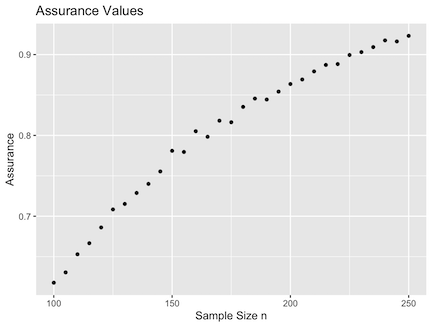Notice that if we input the following parameters into the pwr_freq() function, we observe an approximate overlap between the assurance and power values. n <- seq(100, 250, 5) y1 <- bayesassurance::pwr_freq(n = n, sigsq = 0.265, alt = "greater", alpha = 0.05, theta_0 = 0.15, theta_1 = 0.25) y2 <- assur_vals library(ggplot2) p1 <- ggplot2::ggplot(y1$pwr_table, alpha = 0.5,
aes(x = n, y = Power, color="Power")) +
geom_line(lwd=1.2) + geom_point(data = y2$assurance_table, alpha = 0.5, aes(y = y2$assurance_table$Assurance, color="Assurance"),lwd=1.2) + ggtitle("Power Curve and Assurance Values Overlayed") + xlab("Sample Size n") + ylab("Power/Assurance") p1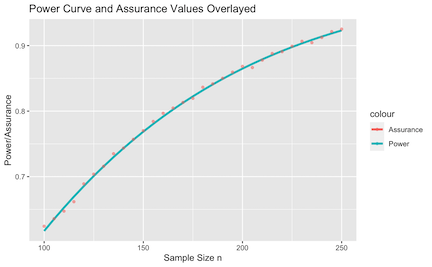Example 2: Use of Linear Contrasts In this next example, we assume $$\beta$$ is a vector of unknown components. We refer to the cost-effectiveness application discussed in O’Hagan et al. 2001 to demonstrate a real-world setting. The application considers a randomized clinical trial that compares the cost-effectiveness of two treatments, where the cost-effectiveness is evaluated using a net monetary benefit measure expressed as $\xi = K(\mu_2 - \mu_1) - (\gamma_2 - \gamma_1),$ where $$\mu_2 - \mu_1$$ and $$\gamma_2 - \gamma_1$$ corresponds to the true differences in treatment efficacy and costs, respectively, between Treatments 1 and 2. The threshold unit cost, $$K$$, represents the maximum price that a health care provider is willing to pay for a unit increase in efficacy. Note that the indices of $$\mu$$ and $$\gamma$$ indicate whether we are looking at Treatment 1 or 2. In this setting, we seek the tenability of $$H: \xi > 0$$, which if true, indicates that Treatment 2 is more cost-effective than Treatment 1. Since the net monetary benefit formula involves assessing the cost and efficacy components conveyed within each of the two treatment groups, we set $$\beta = (\mu_1, \gamma_1, \mu_2, \gamma_2)^{\top}$$, where $$\mu_i$$ and $$\gamma_i$$ denote the efficacy and cost for treatments $$i = 1, 2$$. We therefore set $$u = (-K, 1, K, -1)^{\top}$$ and $$C = 0$$, which lets us evaluate an equivalent form of $$\xi > 0$$ re-expressed $$u^{\top}\beta > 0$$. All other inputs of this application were either specified or deduced from the paper. The following set of inputs specified in the subsequent code chunk should result in an assurance of approximately 0.70 according to O’Hagan et al. 2001. The bayes_sim() returns a similar value, demonstrating that sampling from the posterior yields results similar to those reported in the paper. n = 285 p = 4 K = 20000 # threshold unit cost C <- 0 u <- as.matrix(c(-K, 1, K, -1)) sigsq <- 4.04^2 ## Assign correlation matrices to analysis and design stage priors Vbeta_a_inv <- matrix(rep(0, p^2), nrow = p, ncol = p) Vbeta_d <- (1 / sigsq) * matrix(c(4, 0, 3, 0, 0, 10^7, 0, 0, 3, 0, 4, 0, 0, 0, 0, 10^7), nrow = 4, ncol = 4) tau1 <- tau2 <- 8700 sig <- sqrt(sigsq) Vn <- matrix(0, nrow = n*p, ncol = n*p) Vn[1:n, 1:n] <- diag(n) Vn[(2*n - (n-1)):(2*n), (2*n - (n-1)):(2*n)] <- (tau1 / sig)^2 * diag(n) Vn[(3*n - (n-1)):(3*n), (3*n - (n-1)):(3*n)] <- diag(n) Vn[(4*n - (n-1)):(4*n), (4*n - (n-1)):(4*n)] <- (tau2 / sig)^2 * diag(n) ## Assign mean parameters to analysis and design stage priors mu_beta_d <- as.matrix(c(5, 6000, 6.5, 7200)) mu_beta_a <- as.matrix(rep(0, p)) set.seed(10) assur_vals <- bayesassurance::bayes_sim(n = 285, p = 4, u = as.matrix(c(-K, 1, K, -1)), C = 0, Xn = NULL, Vbeta_d = Vbeta_d, Vbeta_a_inv = Vbeta_a_inv, Vn = Vn, sigsq = 4.04^2, mu_beta_d = as.matrix(c(5, 6000, 6.5, 7200)), mu_beta_a = as.matrix(rep(0, p)), alt = "greater", alpha = 0.05, mc_iter = 10000) assur_vals$assur_val
#>  "Assurance: 0.724"

## 1.2bayes_sim() Special Case: Longitudinal Design

We demonstrate an additional setting embedded in the bayes_sim() function tailored to longitudinal data. Referring back to the linear regression model discussed in the general framework, we can construct a longitudinal model that utilizes the same linear regression form, where $y = X_n\beta + \epsilon_n.$

Consider a group of subjects with an equal number of repeated measures at equally-spaced time points, i.e. a balanced longitudinal study. These subjects can be characterized by $y_{ij} = \beta_{0i} + \beta_{1i}t_{ij} + \epsilon_{ij},$ where $$y_{ij}$$ denotes the $$j^{th}$$ observation of subject $$i$$ at time $$t_{ij}$$, $$\beta_{0i}$$ and $$\beta_{1i}$$ respectively denote the intercept and slope terms for subject $$i$$, and $$\epsilon_{ij}$$ is an error term characterized by $$\epsilon \sim N(0, \sigma^2_i)$$.

In a simple case where we only have two subjects, each with $$n$$ repeated measures, we can individually express the observations as \begin{align*} y_{11} &= \beta_{01} + \beta_{11}t_{11} + \epsilon_{11}\\ &\vdots\\ y_{1n} &= \beta_{01} + \beta_{11}t_{1n} + \epsilon_{1n}\\ y_{21} &= \beta_{02} + \beta_{12}t_{21} + \epsilon_{21}\\ &\vdots\\ y_{2n} &= \beta_{02} + \beta_{12}t_{2n} + \epsilon_{2n}. \end{align*} The model can also be expressed cohesively using matrices, $\begin{gather} \underbrace{\begin{pmatrix} y_{11} \\ \vdots \\ y_{1n} \\ y_{21} \\ \vdots\\ y_{2n} \end{pmatrix}}_{y} = \underbrace{ \begin{pmatrix} 1 & 0 & t_{11} & 0\\ \vdots & \vdots & \vdots & \vdots \\ 1 & 0 & t_{1n} & 0\\ 0 & 1 & 0 & t_{21}\\ \vdots & \vdots & \vdots & \vdots \\ 0 & 1 & 0 & t_{2n} \end{pmatrix} }_{X_n} \underbrace{ \begin{pmatrix} \beta_{01}\\ \beta_{02}\\ \beta_{11}\\ \beta_{12} \end{pmatrix} }_{\beta} + \underbrace{ \begin{pmatrix} \epsilon_{11}\\ \vdots\\ \epsilon_{1n}\\ \epsilon_{21}\\ \vdots\\ \epsilon_{2n} \end{pmatrix} }_{\epsilon_n} \end{gather}$ bringing us back to the linear regression model form.

Example 3: Longitudinal Setting

The following example uses similar parameter settings as the previous cost-effectiveness example along with longitudinal specifications. We assume two subjects and want to test whether the growth rate of subject 1 is greater than that of subject 2. This could have either positive or negative implications depending on the measurement scale.

Assigning an appropriate linear contrast lets us evaluate this under the general expression, $$u^{\top}\beta > C$$, where $$u = (1, -1, 1, -1)^{\top}$$ and $$C = 0$$. The timepoints are arbitrarily chosen to be 10 through 120- this could be days, months, or years depending on the context of the problem. The number of repeated measurements per subject to be tested includes values 10 through 100 in increments of 5. This indicates that we are evaluating the assurance for 19 study designs in total. $$n = 10$$ divides the specified time interval into 10 evenly-spaced timepoints between 10 and 120.

For a more complicated study design consisting of more than two subjects that are divided into two treatment groups, the user could consider testing if the mean growth rate is higher in the first treatment group versus the second, e.g. if we have three subjects per treatment group, the linear contrast could be set as $$u = (0, 0, 0, 0, 0, 0, 1/3, 1/3, 1/3, -1/3, -1/3, -1/3)^{\top}$$.

n <- seq(10, 100, 5)
ids <- c(1,2)
sigsq <- 100
Vbeta_a_inv <- matrix(rep(0, 16), nrow = 4, ncol = 4)
Vbeta_d <- (1 / sigsq) * matrix(c(4, 0, 3, 0, 0, 6, 0, 0, 3, 0, 4, 0, 0, 0, 0, 6),
nrow = 4, ncol = 4)

assur_out <- bayes_sim(n = n, p = NULL, u = c(1, -1, 1, -1), C = 0, Xn = NULL,
Vbeta_d = Vbeta_d, Vbeta_a_inv = Vbeta_a_inv,
Vn = NULL, sigsq = 100,
mu_beta_d = as.matrix(c(5, 6.5, 62, 84)),
mu_beta_a = as.matrix(rep(0, 4)), mc_iter = 5000,
alt = "two.sided", alpha = 0.05, longitudinal = TRUE, ids = ids,
from = 10, to = 120)
head(assur_out$assurance_table) Observations.per.Group..n. Assurance 10 0.7032 15 0.8114 20 0.8870 25 0.9260 30 0.9494 35 0.9654 assur_out$assurance_plot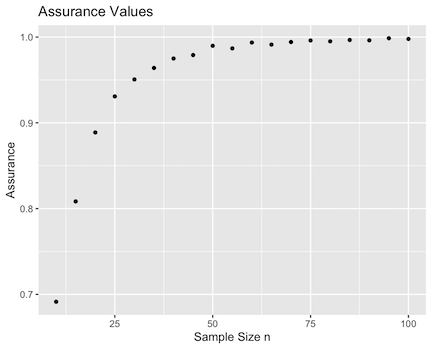# 2 The bayes_sim_unbalanced() Function

The bayes_sim_unbalanced() function relies on the same analysis and design stage framework as bayes_sim() but lets users pass in unequal pairs of sample sizes to determine the assurance of achieving the analysis objective for a multi-sample study design characterized by two distinct sample sizes (e.g. two-sample design with different sized groups, four-sample design with two groups having the same sample size while the other two groups have a different sample size). This is unlike the bayes_sim() function that determines the assurance solely for balanced study designs.

The bayes_sim_unbalanced() function is very similar to bayes_sim() in terms of parameter specifications. There are a few noteworthy exceptions that are listed below.

1. n1: first sample size (vector or scalar). Note that a vector can only be passed in when Xn = NULL. If users choose to specify their own design matrix, n1 must be a scalar as the specified Xn would correspond to a single study design for a specific sample size.
2. n2: second sample size (vector or scalar). Note that a vector can only be passed in when Xn = NULL. If users choose to specify their own design matrix, n2 must be a scalar as the specified Xn would correspond to a single study design for a specific sample size.
3. repeats: an integer giving the number of times to repeat c(n1, n2). Applicable for study designs that have more than two explanatory variables containing the same sample sizes specified in n1 and n2. Set to repeats = 1 by default.
4. surface_plot: logical parameter that specifies whether user wishes to view a contour plot. when set to TRUE, and n1 and n2 are vectors, a contour plot (i.e. heat map) showcasing assurances obtained for unique combinations of n1 and n2 is produced.

The resulting outputs are as follows:

1. assurance_table: table of sample size and corresponding assurance values
2. contourplot: contour map of assurance values if surface_plot = TRUE
3. mc_samples: number of Monte Carlo samples that were generated for evaluation

## 2.1 Examples

Example 1: Simple Example

Similar to bayes_sim(), we let Xn = NULL to allow our function to automatically generate a design matrix using bayesassurance::gen_Xn(), which is discussed in a later section. Note that n1 and n2 can each take a vector of equal length, where a vector of assurance values will be returned corresponding to the distinct pairs of n1 and n2.

n1 <- seq(20, 75, 5)
n2 <- seq(50, 160, 10)

set.seed(100)
assur_out <- bayes_sim_unbalanced(n1 = n1, n2 = n2, repeats = 1, u = c(1, -1),
C = 0, Xn = NULL, Vbeta_d = matrix(c(50, 0, 0, 10),nrow = 2, ncol = 2),
Vbeta_a_inv = matrix(rep(0, 4), nrow = 2, ncol = 2),
Vn = NULL, sigsq = 100,  mu_beta_d = c(1.17, 1.25),
mu_beta_a = c(0, 0), alt = "two.sided", alpha = 0.05, mc_iter = 5000,
surface_plot = TRUE)
## Outputs
head(assur_out$assurance_table) n1 n2 Assurance 20 50 0.9424 25 60 0.9508 30 70 0.9580 35 80 0.9600 40 90 0.9610 45 100 0.9642 assur_out$contourplot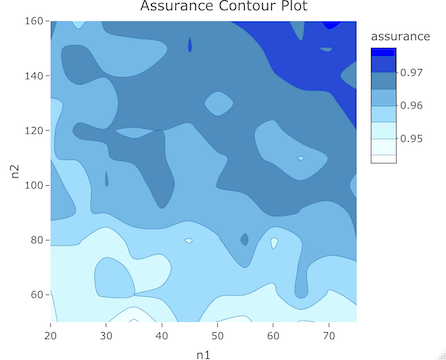Example 2: Cost-effectiveness Example

We revisit the cost-effectiveness example demonstrated in the bayes_sim() discussion. In this example, we need to make use of the repeats parameter as the two sample sizes we are defining correspond to four explanatory variables, i.e. two sets of efficacy and cost measurements pertaining to Treatments 1 and 2.

n1 <- c(4, 5, 15, 25, 30, 100, 200)
n2 <- c(8, 10, 20, 40, 50, 200, 250)

mu_beta_d <- as.matrix(c(5, 6000, 6.5, 7200))
mu_beta_a <- as.matrix(rep(0, 4))
K = 20000 # threshold unit cost
C <- 0
u <- as.matrix(c(-K, 1, K, -1))
sigsq <- 4.04^2
Vbeta_a_inv <- matrix(rep(0, 16), nrow = 4, ncol = 4)
Vbeta_d <- (1 / sigsq) * matrix(c(4, 0, 3, 0, 0, 10^7, 0, 0, 3, 0, 4, 0, 0, 0, 0, 10^7),
nrow = 4, ncol = 4)

set.seed(12)
assur_out <- bayes_sim_unbalanced(n1 = n1, n2 = n2, repeats = 2, u = as.matrix(c(-K, 1, K, -1)),
C = 0, Xn = NULL, Vbeta_d = Vbeta_d,
Vbeta_a_inv = Vbeta_a_inv,
Vn = NULL, sigsq = 4.04^2,
mu_beta_d = as.matrix(c(5, 6000, 6.5, 7200)),
mu_beta_a = as.matrix(rep(0, 4)), alt = "greater",
alpha = 0.05, mc_iter = 5000, surface_plot = TRUE)

View the outputs.

head(assur_out$assurance_table) n1 n2 Assurance 4 8 0.1468 5 10 0.1692 15 20 0.3184 25 40 0.4080 30 50 0.4322 100 200 0.6280 assur_out$contourplot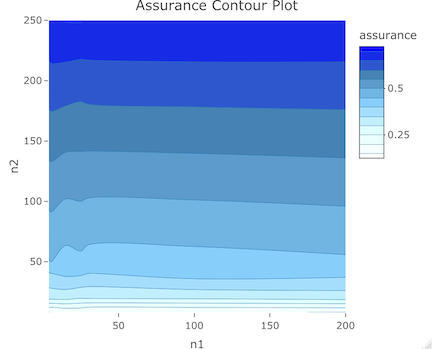# 3 The bayes_sim_unknownvar() Function

Similar to the bayes_sim() function, the bayes_sim_unknownvar() function takes in a set of inputs and returns the approximate Bayesian assurance by determining the proportion of MC samples that meet the analysis stage objective. The bayes_sim_unknownvar() function is used when the variance $$\sigma^2$$ is unknown. Hence, the simulation itself relies on an additional step that involves generating a $$\sigma^2$$ prior to evaluating the analysis objective.

Analysis Stage

Our region of interest corresponding to our analysis objective still remains as $$A_{\alpha}(u, \beta, C) = \left\{y: P\left(u^{\top}\beta \leq C | y\right) < \alpha\right\}$$ when deciding whether or not we are in favor of $$H$$. To implement this in a simulation setting, we rely on iterative sampling for both $$\beta$$ and $$\sigma^2$$ to estimate the assurance. We specify analysis priors $$\beta | \sigma^2 \sim N(\mu_{\beta}^{(a)}, \sigma^2V_{\beta}^{(a)})$$ and $$\sigma^{2} \sim IG(a^{(a)}, b^{(a)})$$, where the superscripts $$(a)$$ signify analysis priors.

We had previously derived the posterior distribution of $$\beta$$ expressed as $$p(\beta | y, \sigma^{2}) = N(\beta | M_nm_n, \sigma^{2}M_n)$$, where $$M_n = (V_\beta^{-1(a)} + X^{\top} V_n^{-1} X)^{-1}$$ and $$m_n = V_\beta^{-1(a)}\mu_\beta^{(a)} + X^{\top}V_n^{-1}y$$. The posterior distribution of $$\sigma^{2}$$ is obtained by integrating out $$\beta$$ from the joint posterior distribution of $$\{\beta, \sigma^2\}$$, which yields $\begin{equation}\label{eq: postbeta} \begin{split} p(\sigma^2| y) &\propto IG(\sigma^2| a^{(a)},b^{(a)}) \times \int N(\beta | \mu_{\beta}, \sigma^2 V_{\beta}) \times N(y | X\beta, \sigma^2 V_{n}) d\beta\\ &\propto \left(\frac{1}{\sigma^2}\right)^{a^{(a)}+ \frac{n}{2} + 1}\exp\left\{-\frac{1}{\sigma^2}\left(b^{(a)} + \frac{c^{\ast}}{2}\right)\right\}\;. \end{split} \end{equation}$ Therefore, $$p(\sigma^2| y) = IG\left(\sigma^2| a^{\ast}, b^{\ast}\right)$$, where $$a^{\ast} = a^{(a)} + \frac{n}{2}$$ and $$b^{\ast} = b^{(a)} + \frac{c^{\ast}}{2} = b^{(a)} + \frac{1}{2} \left\{\mu_{\beta}^{\top (a)} V_{\beta}^{-1(a)}\mu_{\beta}^{(a)} + y^{\top}V_n^{-1}y - m_n^{\top}M_nm_n\right\}$$.

Design Stage

Recall the design stage objective aims to identify sample size $$n$$ that is needed to attain the assurance level specified by the investigator. Similar to Section~$$\ref{subsec: ohagan_stevens_linear_regression_known_sigma}$$ we will need the marginal distribution of $$y$$ with priors placed on both $$\beta$$ and $$\sigma^2$$. We denote these design priors as $$\beta^{(d)}$$ and $$\sigma^{2(d)}$$, respectively, to signify that we are working within the design stage. Derivation steps are almost identical to those outlined in bayes_sim() for the known $$\sigma^2$$ case. With $$\sigma^{2(d)}$$ now treated as an unknown parameter, the marginal distribution of $$y$$, given $$\sigma^{2(d)}$$, under the design prior is derived from $$y = X_n\beta^{(d)} + e_n$$, $e_n N(0, ^{2(d)} V_n)$, $$\beta^{(d)} = \mu_{\beta}^{(d)} + \omega; \quad \omega \sim N(0, \sigma^{2(d)} V_{\beta}^{(d)})$$, where $$\beta^{(d)}\sim N(\mu_{\beta}^{(d)},\sigma^{2(d)} V_{\beta}^{(d)})$$ and $$\sigma^{2(d)} \sim IG(a^{(d)}, b^{(d)})$$. Substituting $$\beta^{(d)}$$ into $$y$$ gives us $$y = X_n\mu_{\beta}^{(d)} + (X_n\omega + e_n)$$ such that $$X_n\omega + e_n \sim N(0, \sigma^{2(d)}(V_n + X_n V_{\beta}^{(d)} X_n^{\top}))$$. The marginal distribution of $$p(y| \sigma^{2(d)})$$ is $\begin{equation}\label{eq:marg_yn} y | \sigma^{2(d)} \sim N(X_n \mu_{\beta}^{(d)}, \sigma^{2(d)} V_n^{*}); \quad V_n^{*} = X_n V_{\beta}^{(d)} X_n^{\top} + V_n. \end{equation}$ Equation~$$\eqref{eq:marg_yn}$$ specifies our data generation model for ascertaining sample size.

Summary

In the design stage, we draw $$\sigma^{2(d)}$$ from $$IG(a_\sigma^{(d)}, b_\sigma^{(d)})$$ and generate the data from our sampling distribution from $$\eqref{eq:marg_yn}$$, $$y \sim N(X\mu_{\beta}^{(d)}, \sigma^{2(d)}(XV_{\beta}^{(d)}X^{\top} + V_n))$$. For each such data set, $$\{y, X_n\}$$, we perform Bayesian inference for $$\beta$$ and $$\sigma^2$$. Here, we draw $$J$$ samples of $$\beta$$ and $$\sigma^2$$ from their respective posterior distributions and compute the proportion of these $$J$$ samples that satisfy $$u^{\top} \beta_j > C$$. If the proportion exceeds a certain threshold $$1-\alpha$$, then the analysis objective is met for that dataset. The above steps for the design and analysis stage are repeated for $$R$$ datasets and the proportion of the $$R$$ datasets that meet the analysis objective, i.e., deciding in favor of $$H$$, correspond to the Bayesian assurance.

## 3.1 Example

Taking the exact same inputs as those in the first example of bayes_sim(), we modify the inputs and perform the simulation using bayes_sim_unknownvar() instead.

n <- seq(100, 250, 5)
assur_vals <- bayesassurance::bayes_sim(n, p = 1, u = 1, C = 0.15, Xn = NULL,
Vbeta_d = 1e-8, Vbeta_a_inv = 0,
Vn = NULL, sigsq = 0.265,
mu_beta_d = 0.25, mu_beta_a = 0,
alpha = 0.05, mc_iter = 10000)

assur_vals <- bayesassurance::bayes_sim_unknownvar(n, p = 1, u = 1,
C = 0.15, R = 150, Xn = NULL, Vn = NULL,
Vbeta_d = 1e-8, Vbeta_a_inv = 0,
mu_beta_d = 0.25, mu_beta_a = 0,
a_sig_a = 0.1, b_sig_a = 0.1,
a_sig_d = 0.1, b_sig_d = 0.1,
alpha = 0.05, mc_iter = 5000)

# 4 Generating the Design Matrix Using gen_Xn()

All simulation-based functions contained in the package do not require users to specify their own design matrix $$X_n$$. Users have the option of setting Xn = NULL, which prompts the function to construct a default design matrix using bayesassurance::gen_Xn(). The resulting design matrix depends on the sample size parameter n being passed into bayes_sim(). Each unique sample size contained in n results in a distinct design matrix. The column dimension of $$X_n$$ corresponds to the number of groups being assessed, denoted by parameter p. Each column is comprised of ones vectors that run diagonally across the matrix with lengths that correspond to the sample size value.

The following examples showcase the general functionality of gen_Xn() as well as how gen_Xn() is specifically used within bayes_sim().

Example 1

As a general example, suppose we pass in a vector of length 4 into gen_Xn(). The function will interpret this as a study design consisting of 4 explanatory variables with unequal observed frequencies of 1, 2, 3, and 4. This will result in $$X_n$$ having 4 columns containing ones vectors of lengths 1, 2, 3, and 4 respectively.

n <- c(1,2,3,4)
bayesassurance::gen_Xn(n)
#>       [,1] [,2] [,3] [,4]
#>  [1,]    1    0    0    0
#>  [2,]    0    1    0    0
#>  [3,]    0    1    0    0
#>  [4,]    0    0    1    0
#>  [5,]    0    0    1    0
#>  [6,]    0    0    1    0
#>  [7,]    0    0    0    1
#>  [8,]    0    0    0    1
#>  [9,]    0    0    0    1
#> [10,]    0    0    0    1

Example 2

The bayes_sim() function assumes a balanced study design such that the $$p$$ explanatory variables have an equal number of observations. When a vector of sample sizes is passed into bayes_sim() for parameter $$n$$, each value within $$n$$ corresponds to a separate study design that results in a different design matrix. By specifying $$p$$, the resulting $$X_n$$ will have $$p$$ columns with ones vectors of equal length running diagonally across the matrix.

n <- 3
p <- 4
bayesassurance::gen_Xn(rep(n, p))
#>       [,1] [,2] [,3] [,4]
#>  [1,]    1    0    0    0
#>  [2,]    1    0    0    0
#>  [3,]    1    0    0    0
#>  [4,]    0    1    0    0
#>  [5,]    0    1    0    0
#>  [6,]    0    1    0    0
#>  [7,]    0    0    1    0
#>  [8,]    0    0    1    0
#>  [9,]    0    0    1    0
#> [10,]    0    0    0    1
#> [11,]    0    0    0    1
#> [12,]    0    0    0    1

It is actually encouraged for users to set Xn = NULL as this will greatly facilitate the process of determining the assurances for multiple sample sizes at once. This saves the trouble of having users define their own separate design matrices for each sample size.

# 5 Generating the Design Matrix in a Time-Based Setting Using gen_Xn_longitudinal()

When working in the longitudinal setting, additional parameters need to be specified prior to running bayes_sim(). These include

1. longitudinal: when set to TRUE, this informs bayes_sim() that the simulation will be based in a longitudinal setting. If Xn = NULL, the function will construct a design matrix using inputs that correspond to a balanced longitudinal study design.
2. ids: vector of unique subject ids
3. from: start time of repeated measures for each subject
4. to: end time of repeated measures for each subject

The parameter n plays a vital role in this setting as its value(s) correspond to the number of repeated measures each subject has. Referring to the sequence generating function, seq(), in R’s base package, the time points for each subject are determined as seq(from = from, to = to, length.out = n).

The longitudinal setting in bayes_sim() implicitly relies on the bayesassurance::gen_Xn_longitudinal() function to construct its design matrices. The user does not need to explicitly call on this function when setting Xn = NULL. Each unique sample size contained in n corresponds to a distinct study design.

The next example shows how gen_Xn_longitudinal() is used. $$X_n$$ takes the form of the design matrix highlighted in the linear regression model discussed in the previous section, comprised of ones vectors in the first set of columns and vectors of time points in the second half. When Xn = NULL and longitudinal = TRUE, the bayes_sim() function will automatically generate a design matrix using this feature.

** Example **

ids <- c(1,2,3,4)
gen_Xn_longitudinal(ids, from = 1, to = 10, num_repeated_measures = 4)
#>       [,1] [,2] [,3] [,4] [,5] [,6] [,7] [,8]
#>  [1,]    1    0    0    0    1    0    0    0
#>  [2,]    1    0    0    0    4    0    0    0
#>  [3,]    1    0    0    0    7    0    0    0
#>  [4,]    1    0    0    0   10    0    0    0
#>  [5,]    0    1    0    0    0    1    0    0
#>  [6,]    0    1    0    0    0    4    0    0
#>  [7,]    0    1    0    0    0    7    0    0
#>  [8,]    0    1    0    0    0   10    0    0
#>  [9,]    0    0    1    0    0    0    1    0
#> [10,]    0    0    1    0    0    0    4    0
#> [11,]    0    0    1    0    0    0    7    0
#> [12,]    0    0    1    0    0    0   10    0
#> [13,]    0    0    0    1    0    0    0    1
#> [14,]    0    0    0    1    0    0    0    4
#> [15,]    0    0    0    1    0    0    0    7
#> [16,]    0    0    0    1    0    0    0   10